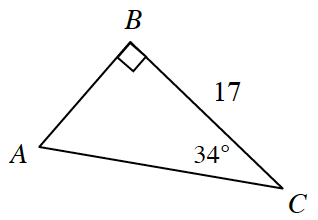### Home > INT2 > Chapter 6 > Lesson 6.2.4 > Problem6-87

6-87.

Determine the area of the triangle at right. Show all work.Use the tangent ratio to solve for $AB$. Refer to the Math Notes box in Lesson 4.2.2 for help.

$\text{tan}(34^\circ) = \frac{\it{AB}}{17}$